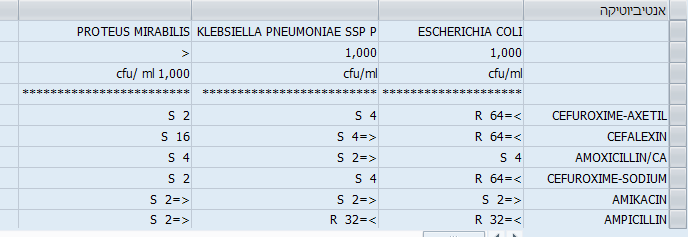OHDSI Home | Forums | Wiki | Github# Unit_concept_id in measurements

Hi,
We would appreciate help in mapping unit concept to out measurement table - lab results
we have colony forming units per mililiter [CFU]/ml with unit concept id of 9423
on the other hane we have 1000 colony forming units per mililiter - for this unit I am unable to find a concept ID.
how would this be mapped?
appreciate help on this matter
Robyn

Not sure what the problem is. If it is 1 colony forming unit per ml it is 1. If it is 1000 it is 1000. What are you up to?

I think the problem is ETL can’t convert the numbers that are property of the unit and not the property of the value.

The agreed approach is:

1. We create “source” units for every needed multiplication of the actual unit.
2. We leave them Standard unless we mapped them to the respective actual units with the conversion factors.
3. ETL can use the new Standard unit now and start doing the value conversion once the unit mapping is done on the vocabulary end.

Makes sense?as we can see the column in the attached figure “proteus mirabilis” displays the unit as cfu/ml 1,000.
My inclination is to regard it as cfu/ml

t